# (Machine|Statistical) Learning - (Target|Learned|Outcome|Dependent|Response) (Attribute|Variable) (Y|DV)

An (outcome|dependent) variable is a measure that we want to predict.

There is two type of outcome variables:

A dependent variable (DV) is measured during the experimentation in contrast with independent variable (IV) that describes:

• the characteristics of the group (such as name)
• and/of the experimentation (such as the steps: before, after).

The target Attribute in your build data of a supervised model is the attribute that you try to predict.

The target attribute is also called:

• Outcome measurement (Y)
• Outcome variable (Y)
• Output variable
• Label
• Dependent variable (DV),
• Response variable. (It's a statistic term when groups respond to a treatment)
• Responding variable
• Regressand
• Explained variable
• Experimental variable
• Output variable
• Criterion Variable
• Unobserved variable
• Predicted variable
• Measured variable

When the target attribute is:

In the test data, it will contain values with known outcomes in order to measure the performance of the model.

In the scoring data, it will contains the results when the model is applied.

Clustering, feature extraction, association, and anomaly detection models do not use a target because they are unsupervised function.

When the target is predicted, we get an predicted target and you can find the following notations in formulas.

• $Y$ is the original target score from the training data (ie collected)
• $\hat{Y}$ is the predicted score from the model.

## Documentation / Reference

Recommended PagesData Mining - (two class|binary) classification problem (yes/no, false/true)

Binary classification is used to predict one of two possible outcomes. A two class problem (binary problem) has possibly only two outcomes: “yes or no” “success” or “failure” and is...(Machine|Statistical) Learning - (Predictor|Feature|Regressor|Characteristic) - (Independent|Explanatory) Variable (X)

A Independent variable is a variable used in supervised analysis in order to predict an outcome variable. It's also known as: Predictor Input variable, Regressors, Explanatory variable, CovariateCovariates...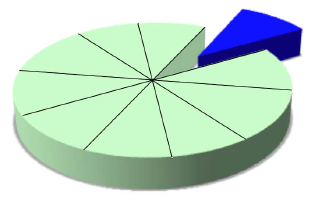(Statistics|Data Mining) - (K-Fold) Cross-validation (rotation estimation)

Cross-validation, sometimes called rotation estimation is a resampling validation technique for assessing how the results of a statistical analysis will generalize to an independent new data set. This...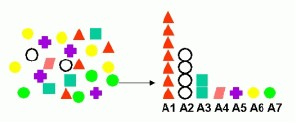Data Mining - Attribute (Importance|Selection) - Affinity Analysis

Attribute importance is a supervised function that identifies and ranks the attributes that are most important in predicting a target attribute. Oracle Data Mining does not support the scoring operation...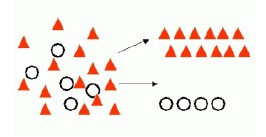Data Mining - (Classifier|Classification Function)

A classifier is a Supervised function (machine learning tool) where the learned (target) attribute is categorical (“nominal”) in order to classify. It is used after the learning process to classify...Data Mining - (Class|Category|Label) Target

A class is the category for a classifier which is given by the target. The number of class to be predicted define the classification problem. A class is also known as a label. Labeled...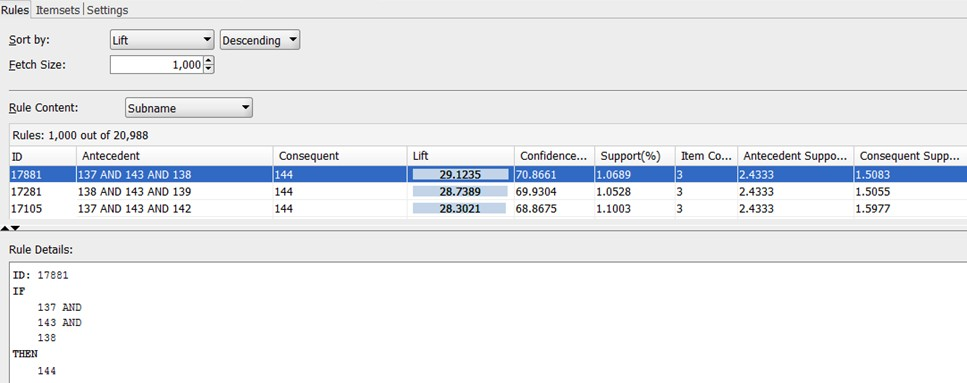Data Mining - (Decision) Rule

Some forms of predictive data mining generate rules that are conditions that imply a given outcome. Rules are if-then-else expressions; they explain the decisions that lead to the prediction. They...Data Mining - (Discriminative|conditional) models

Discriminative models, also called conditional models, are a class of models used in machine learning for modeling the dependence of an unobserved variable y on an observed variable x. Discriminative...Data Mining - (Function|Model)

The model is the function, equation, algorithm that predicts an outcome value from one of several predictors. During the training process, the models are build. A model uses a logic and one of several...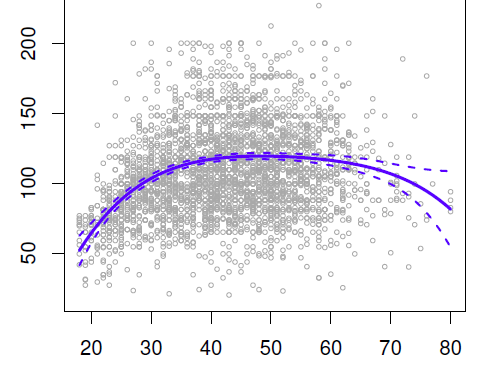Data Mining - (Global) Polynomial Regression (Degree)

polynomials regression Although polynomials are easy to think of, splines are much better behaved and more local. With polynomial regression, you create new variables that are just transformations...